# Samacheer Kalvi 10th Maths Solutions Chapter 5 Coordinate Geometry Ex 5.5

## Tamilnadu Samacheer Kalvi 10th Maths Solutions Chapter 5 Coordinate Geometry Ex 5.5

Question 1.
The area of triangle formed by the points (-5, 0), (0, -5) and (5, 0) is
(1) 0 sq. units
(2) 25 sq.units
(3) 5 sq. units
(4) none of these
Solution:
(2) 25 sq. units
Hint: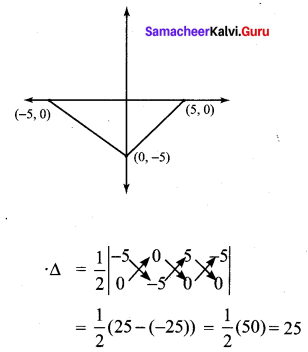Question 2.
A man walks near a wall, such that the distance between him and the wall is 10 units.
Consider the wall to be the Y axis. The path travelled by the man is
(1) x = 10
(2) y = 10
(3) x = 0
(4) y = 0
Solution:
(1) x = 10
Hint: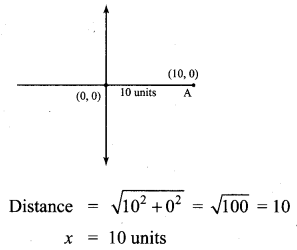Question 3.
The straight line given by the equation x = 11 is
(1) parallel to X axis
(2) parallel to Y axis
(3) passing through the origin
(4) passing through the point (0,11)
Solution:
(2) Parallel to y axis
Hint: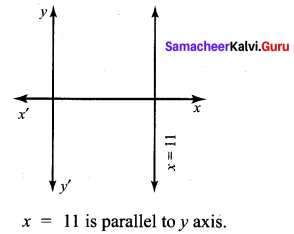Question 4.
If (5, 7), (3, p) and (6, 6) are collinear, then the value of p is
(1) 3
(2) 6
(3) 9
(4) 12
Solution:
(3) 9
If (5, 7), (3, p) and (6, 6) are collinear ∆ = 0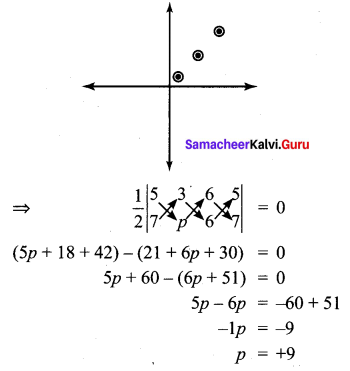Question 5.
The point of intersection of 3x – y = 4 and x + y = 8 is
(1) (5, 3)
(2) (2, 4)
(3) (3, 5)
(4) (4, 4)
Solution:
(3) (3, 5)]
Hint: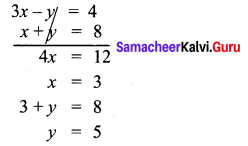∴ Point of intersection is (3, 5)Question 6.
The slope of the line joining (12, 3), (4, a) is $$\frac{1}{8}$$. The value of ‘a’ is
(1) 1
(2) 4
(3) -5
(4) 2
Solution:
(4) 2
Hint: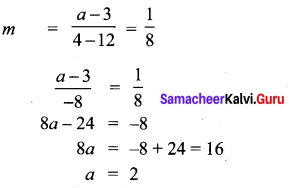Question 7.
The slope of the line which is perpendicular to a line joining the points (0, 0) and (-8, 8) is
(1) -1
(2) 1
(3) $$\frac{1}{3}$$
(4) -8
Solution:
(2) 1
Hint:
Slope of the line joining the points (0, 0) and (-8, 8) is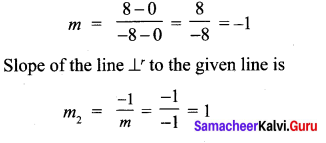Question 8.
If slope of the line PQ is $$\frac{1}{\sqrt{3}}$$ then slope of the perpendicular bisector of PQ is
(1) $$\sqrt{3}$$
(2) $$-\sqrt{3}(3)$$
(3) $$\frac{1}{\sqrt{3}}$$
(4) 0
Solution:
(2) $$-\sqrt{3}(3)$$

Question 9.
If A is a point on the Y axis whose ordinate is 8 and B is a point on the X axis whose abscissa is 5 then the equation of the line AB is
(1) 8x + 5y = 40
(2) 8x – 5y = 40
(3) x = 8
(4) y = 5
Solution:
(1) 8x + 5y = 40
Hint: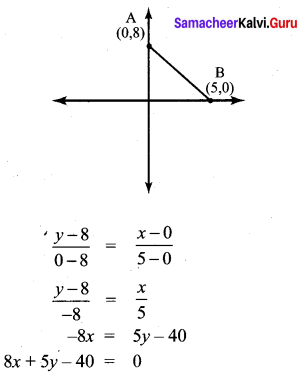Question 10.
The equation of a line passing through the origin and perpendicular to the line 7x – 3y + 4 = 0 is
(1) 7x – 3y + 4 = 0
(2) 3x – 7y + 4 = 0
(3) 3x + 7y = 0
(4) 7x – 3y = 0
Solution:
(3) 3x + 7y = 0
Hint: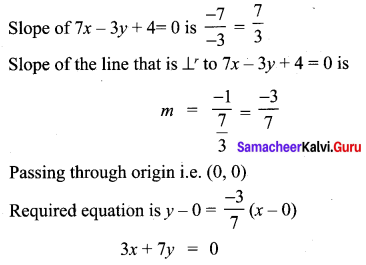Question 11.
Consider four straight lines
(i) l1 : 3y = 4x + 5
(ii) l2: 4y = 3x – 1
(iii) l3: 4y = 3x = 7
(iv) l4 : 4x + 3y = 2
Which of the following statement is true ?
(1) l1 and l2 are perpendicular
(2) l1 and l4 are parallel
(3) l2 and l4 are perpendicular
(4) l2 and l3 are parallel
Solution:
(3) l2 and l4 are perpendicular
Hint: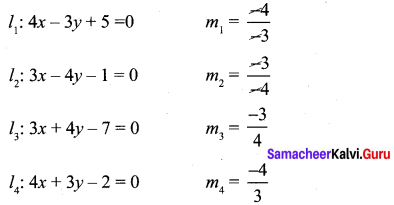Question 12.
A straight line has equation 8y = 4x + 21. Which of the following is true
(1) The slope is 0.5 and the y intercept is 2.6
(2) The slope is 5 and they intercept is 1.6
(3) The slope is 0.5 and the y intercept is 1.6
(4) The slope is 5 and they intercept is 2.6
Solution:
(1) The slope is 0.5 and the y intercept is 2.6
Hint: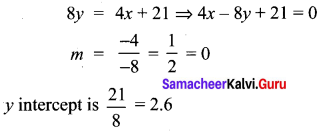Question 13.
When proving that a quadrilateral is a trapezium, it is necessary to show ………………
(1) Two sides are parallel.
(2) Two parallel and two non-parallel sides.
(3) Opposite sides are parallel.
(4) All sides are of equal length.
(2) Two parallel and two non-parallel sides.Question 14.
When proving that a quadrilateral is a parallelogram by using slopes you must find
(1) The slopes of two sides
(2) The slopes of two pair of opposite sides
(3) The lengths of all sides
(4) Both the lengths and slopes of two sides
Solution:
(2) The slopes of two pair of opposite sides

Question 15.
(2, 1) is the point of intersection of two lines.
(1) x – y – 3 = 0; 3x – y – 7 = 0
(2) x + y = 3; 3x + y = 7
(3) 3x + y = 3; x + y = 7
(4) x + 3y – 3 = 0; x – y – 7 = 0
(2) x + y = 3; 3x + y = 7
Hint:
Substitute the value of x = 2 and y = 1 in the given equation.
(1) ⇒ x – y – 3 = 0
2 – 1 – 3 = 0
2 – 4 = 0
– 2 ≠ 0
not true

3x – y – 7 = 0
3(2) – 1 – 7 = 0
6 – 8 = 0
-2 ≠ 0
not true

(2) ⇒ x + y = 3
2 + 1 = 3
3 = 3
True

3x + y = 7
3(2) + 1 = 7
6 + 1 = 7
7 = 7
True
∴ (2, 1) is the point of intersection

(3) ⇒ 3x + y = 3
3(2) + 1 = 3
6 + 1 = 3
7 = 3
not true

x + y = 7
2 + 1 = 7
3 = 7
not true

(4) ⇒ x + 3y – 3 = 0
2 + 3 – 3 = 0
5 – 3 = 0
2 ≠ 0
not true

x – y – 7 = 0
2 – 1 – 7 = 0
2 – 8 = 0
-6 ≠ 0
not true